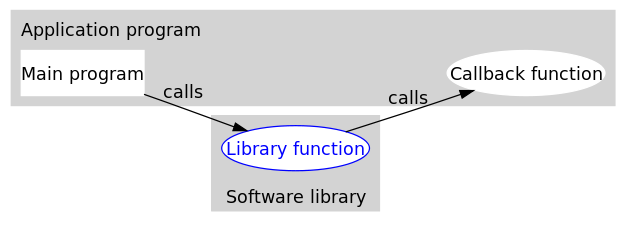# CallBack回调函数`even.py`

``````    #回调函数1
#生成一个2k形式的偶数
def double(x):
return x * 2

#回调函数2
#生成一个4k形式的偶数
return x * 4``````

`callback_demo.py`

``````    from even import *

#中间函数
#接受一个生成偶数的函数作为参数
#返回一个奇数
def getOddNumber(k, getEvenNumber):
return 1 + getEvenNumber(k)

#起始函数，这里是程序的主函数
def main():
k = 1
#当需要生成一个2k+1形式的奇数时
i = getOddNumber(k, double)
print(i)
#当需要一个4k+1形式的奇数时
print(i)
#当需要一个8k+1形式的奇数时
i = getOddNumber(k, lambda x: x * 8)
print(i)

if __name__ == "__main__":
main()``````

``````3
5
9``````

## 本文链接：

https://lod.pub/6.html
1 + 5 =